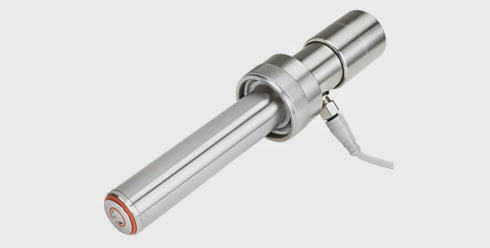### Hydrostatic Tank Level MeasurementKing-Gage pressure transmitter
One of the most proven and reliable methods of continuous tank level monitoring is remotely monitoring hydrostatic pressure.

Hydrostatic pressure is defined as "The pressure exerted by a fluid at equilibrium at a given point within the fluid, due to the force of gravity. Hydrostatic pressure increases in proportion to depth measured from the surface because of the increasing weight of fluid exerting downward force from above.

Pressure transmitters are a simple and accurate choice for measuring liquid level in storage tanks or processing tanks.Typical hydrostatic level application
The principle behind hydrostatic, pressure-based, liquid level measurement is simple since pressure is proportional to the level of liquid multiplied by the specific gravity. The specific gravity of a liquid is the ratio of its own density to the density of water.

Alternatively, level equals the hydrostatic head pressure value divided by the density of the liquid.